Technical Test SSC JE: Electrical Engineering (EE)- 15

# Technical Test SSC JE: Electrical Engineering (EE)- 15

Test Description

## 100 Questions MCQ Test Mock Test Series for SSC JE Electrical Engineering | Technical Test SSC JE: Electrical Engineering (EE)- 15

Technical Test SSC JE: Electrical Engineering (EE)- 15 for Electrical Engineering (EE) 2022 is part of Mock Test Series for SSC JE Electrical Engineering preparation. The Technical Test SSC JE: Electrical Engineering (EE)- 15 questions and answers have been prepared according to the Electrical Engineering (EE) exam syllabus.The Technical Test SSC JE: Electrical Engineering (EE)- 15 MCQs are made for Electrical Engineering (EE) 2022 Exam. Find important definitions, questions, notes, meanings, examples, exercises, MCQs and online tests for Technical Test SSC JE: Electrical Engineering (EE)- 15 below.
Solutions of Technical Test SSC JE: Electrical Engineering (EE)- 15 questions in English are available as part of our Mock Test Series for SSC JE Electrical Engineering for Electrical Engineering (EE) & Technical Test SSC JE: Electrical Engineering (EE)- 15 solutions in Hindi for Mock Test Series for SSC JE Electrical Engineering course. Download more important topics, notes, lectures and mock test series for Electrical Engineering (EE) Exam by signing up for free. Attempt Technical Test SSC JE: Electrical Engineering (EE)- 15 | 100 questions in 100 minutes | Mock test for Electrical Engineering (EE) preparation | Free important questions MCQ to study Mock Test Series for SSC JE Electrical Engineering for Electrical Engineering (EE) Exam | Download free PDF with solutions
 1 Crore+ students have signed up on EduRev. Have you?
Technical Test SSC JE: Electrical Engineering (EE)- 15 - Question 1

### The smallest change in a measured variable to which an instrument will respond is

Technical Test SSC JE: Electrical Engineering (EE)- 15 - Question 2

### Two bulbs marked 200 wtt-250 volts and 100 watt-250 volts are joined in series to 250 volts supply. Power consumed in circuit is

Technical Test SSC JE: Electrical Engineering (EE)- 15 - Question 3

### Hysteresis losses in a d.c. machine are proportional to

Technical Test SSC JE: Electrical Engineering (EE)- 15 - Question 4

Torque/ weight ratio of an instrument indicates

Technical Test SSC JE: Electrical Engineering (EE)- 15 - Question 5

Series reactors are used to

Technical Test SSC JE: Electrical Engineering (EE)- 15 - Question 6

What is the range for a   3(1/2)digit digital voltmeter ?

Technical Test SSC JE: Electrical Engineering (EE)- 15 - Question 7

........ is the region of a transistor which has highest conductivity.

Technical Test SSC JE: Electrical Engineering (EE)- 15 - Question 8

Two coils have self-inductances of 0.09 H and 0.01 H and mutual inductance of 0.015 H. The coefficient of coupling between coils is

Technical Test SSC JE: Electrical Engineering (EE)- 15 - Question 9

Two resistances R1 and R2 give combined resistance of 4.5 ohms when in series and 1 ohm when in parallel. The resistance are

Technical Test SSC JE: Electrical Engineering (EE)- 15 - Question 10

In a star-connected system without neutral grounding, zero-sequence currents are

Technical Test SSC JE: Electrical Engineering (EE)- 15 - Question 11

Sensitivity of a voltmeter is given as

Technical Test SSC JE: Electrical Engineering (EE)- 15 - Question 12

If one of the central springs of a Permanent Magnet Moving Coil ammeter is broken then when connected it will read

Technical Test SSC JE: Electrical Engineering (EE)- 15 - Question 13

The positive sequence current of a transmission line is :

Technical Test SSC JE: Electrical Engineering (EE)- 15 - Question 14

The teeth in the armature of a dc machine are sometimes skewed. This is done to reduce

Technical Test SSC JE: Electrical Engineering (EE)- 15 - Question 15

When a line-to-ground fault occurs, the current in the faulted phase is 100 A. The zero-sequence current in this case will be :

Technical Test SSC JE: Electrical Engineering (EE)- 15 - Question 16

Resistance across A and B in the circuit shown below is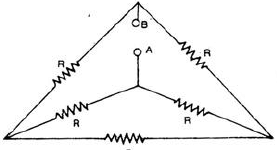Technical Test SSC JE: Electrical Engineering (EE)- 15 - Question 17

In a CRT the highest positive potential is given to

Technical Test SSC JE: Electrical Engineering (EE)- 15 - Question 18

the purpose of the synchronising control in a CRO is to

Technical Test SSC JE: Electrical Engineering (EE)- 15 - Question 19

Power factor of an inductive circuit can be improved by connecting a capacitor to it in

Technical Test SSC JE: Electrical Engineering (EE)- 15 - Question 20

In measurements made using a Q-meter impedance elements should preferably be connected in

Technical Test SSC JE: Electrical Engineering (EE)- 15 - Question 21

Wagner Eearth devices in ac bridge circuits are used for

Detailed Solution for Technical Test SSC JE: Electrical Engineering (EE)- 15 - Question 21

Wagner’s earth devices are used in ac bridge circuits to eliminate the effect of earth capacitance.

Technical Test SSC JE: Electrical Engineering (EE)- 15 - Question 22

When the supply terminals of a dc shunt motor are interchanged

Technical Test SSC JE: Electrical Engineering (EE)- 15 - Question 23

The current of a single-phase load drawn from a 3-phase system has:

Technical Test SSC JE: Electrical Engineering (EE)- 15 - Question 24

Systematic error are

Technical Test SSC JE: Electrical Engineering (EE)- 15 - Question 25

The minimum number of wattmeter (s) required to measure 3-phase 3-wire balanced or unbalance power is

Technical Test SSC JE: Electrical Engineering (EE)- 15 - Question 26

The voltage coil of a single phase house energy meter

Technical Test SSC JE: Electrical Engineering (EE)- 15 - Question 27

Which one of the following methods decreases the error due to connections in a dynamometer type wattmeter ?

Technical Test SSC JE: Electrical Engineering (EE)- 15 - Question 28

If the positive-, negative-and zero-sequence reactances of an element of a power system are 0.3, 0.3 and 0.8 pu respectively, then the element would be a

Technical Test SSC JE: Electrical Engineering (EE)- 15 - Question 29

A separately excited dc generator is feeding a dc shunt motor. If the load torque on the motor is halved approximately

Technical Test SSC JE: Electrical Engineering (EE)- 15 - Question 30

A manganin swamp resistance is connected in series with a moving coil ammeter consisting of a milliameeter and a suitable shunt in order of

Technical Test SSC JE: Electrical Engineering (EE)- 15 - Question 31

If starter is not used dc motor for starting, it will draw a starting current which

Technical Test SSC JE: Electrical Engineering (EE)- 15 - Question 32

The deflecting torque of a moving iron instrument is

Technical Test SSC JE: Electrical Engineering (EE)- 15 - Question 33

If all the sequence voltages at the fault point in a power system are equal, then the fault is a

Technical Test SSC JE: Electrical Engineering (EE)- 15 - Question 34

A set of independent current measurements taken by four observers was recorded as : 117.02 mA, 117.11 mA, 117.08 mA and 117.03 mA. What is the range of error ?

Technical Test SSC JE: Electrical Engineering (EE)- 15 - Question 35

Two coils having equal resistances but different inductances are connected in series. The time constant of the series combination is the

Technical Test SSC JE: Electrical Engineering (EE)- 15 - Question 36

The zero-sequence circuit of the three-phase transformer shown in the figure is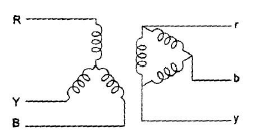Technical Test SSC JE: Electrical Engineering (EE)- 15 - Question 37

The accuracy of Kelvin’s double bridge for the measurement of low resistance is high because the bridge

Technical Test SSC JE: Electrical Engineering (EE)- 15 - Question 38

The octal equivalent of decimal 98 is

Technical Test SSC JE: Electrical Engineering (EE)- 15 - Question 39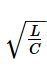has the dimension of

Technical Test SSC JE: Electrical Engineering (EE)- 15 - Question 40

Steady state stability of a power system is improved by

Technical Test SSC JE: Electrical Engineering (EE)- 15 - Question 41

The transistor is usually encapsuled in –

Technical Test SSC JE: Electrical Engineering (EE)- 15 - Question 42

An alternating e.m.f. is applied to a pure inductance such that inductive reactance is 10 W.
If the frequency of a.c. is doubled, the reactance will become

Technical Test SSC JE: Electrical Engineering (EE)- 15 - Question 43

The positive-sequence current for a L-L fault of a 2kV system is 1,400 A, and corresponding current for a L-L-G fault is 2,220 A.

The zero-sequence impedance of the system is

Technical Test SSC JE: Electrical Engineering (EE)- 15 - Question 44

An RLC resonant circuit has a resonance frequency of 1.5 MHz and a bandwidth of 10
kHz. If C = 150 pF, then the effective resistance of the circuit will be

Technical Test SSC JE: Electrical Engineering (EE)- 15 - Question 45

Match List I with List II and select the correct answer using the codes given below the lists: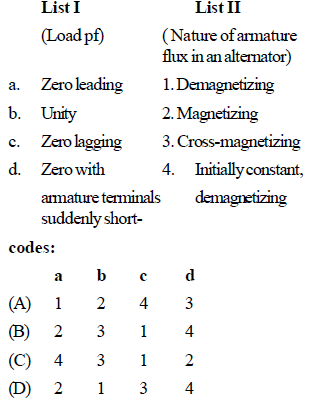Technical Test SSC JE: Electrical Engineering (EE)- 15 - Question 46

For the same voltage drop, increasing the voltage of a distributor n-times.

Technical Test SSC JE: Electrical Engineering (EE)- 15 - Question 47

A single – Phase, 200 V alternator has armature resistance and reactance of 0.8W and 4.94W respectively. The voltage regulation of the alternator at 100 A load at 0.8 leading power factor is

Technical Test SSC JE: Electrical Engineering (EE)- 15 - Question 48

The insulation coordination for UHV lines (above 500 kV) is done base on

Technical Test SSC JE: Electrical Engineering (EE)- 15 - Question 49

A SCIM having a rated slip of 4% on full load has a starting torque the same as full-load torque.The starting current is

Technical Test SSC JE: Electrical Engineering (EE)- 15 - Question 50

Lightning is a huge spark caused by electrical discharge taking place between

Technical Test SSC JE: Electrical Engineering (EE)- 15 - Question 51

A network N ' is dual of network N if

Technical Test SSC JE: Electrical Engineering (EE)- 15 - Question 52

Which of the following is usually not the generating voltage ?

Technical Test SSC JE: Electrical Engineering (EE)- 15 - Question 53

Post-deflection is required in CRO, if input signal frequency is

Technical Test SSC JE: Electrical Engineering (EE)- 15 - Question 54

In a resistance loaded, RC coupled amplifier the D.C. component is blocked by –

Technical Test SSC JE: Electrical Engineering (EE)- 15 - Question 55

An higher scale ammeter is used to measure too low current. The measurement would have low

Technical Test SSC JE: Electrical Engineering (EE)- 15 - Question 56

Dominating harmonics in IM is

Technical Test SSC JE: Electrical Engineering (EE)- 15 - Question 57

A 3-Phase star-connected symmetrical load consumes P watts of power from a balance supply. If the same load is connected in delta to the same supply , the power consumption will be

Technical Test SSC JE: Electrical Engineering (EE)- 15 - Question 58

Dead zone of an instrument is

Technical Test SSC JE: Electrical Engineering (EE)- 15 - Question 59

Which of the following components of a D.C. generator plays vital role for providing direct current of a D.C. generator ?

Technical Test SSC JE: Electrical Engineering (EE)- 15 - Question 60

What is the slope of the line due to1/jW  factor in magnitude part of Bode plot?

Technical Test SSC JE: Electrical Engineering (EE)- 15 - Question 61

For the circuit shown in fig. the meter will read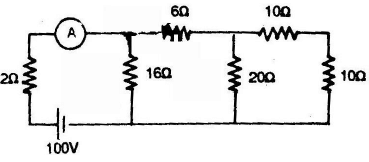Technical Test SSC JE: Electrical Engineering (EE)- 15 - Question 62

The ratio of starting torque to full load torque is least in case of—

Technical Test SSC JE: Electrical Engineering (EE)- 15 - Question 63

An indicating instrument is more sensitive if its torque to weight ratio is

Technical Test SSC JE: Electrical Engineering (EE)- 15 - Question 64

When a capacitor was connected to the terminal of an ohmmeter the pointer indicated a low resistance initially and then slowly came to infinity position. This shows that the capacitor is

Technical Test SSC JE: Electrical Engineering (EE)- 15 - Question 65

Impulse ratios of insulators and lightning arresters should be

Technical Test SSC JE: Electrical Engineering (EE)- 15 - Question 66

For complete protection of a 3-phase transmission line we require

Technical Test SSC JE: Electrical Engineering (EE)- 15 - Question 67

Hopkinson’s test is conducted at—

Technical Test SSC JE: Electrical Engineering (EE)- 15 - Question 68

The difference between the indicated value and the true value of a quantity is

Technical Test SSC JE: Electrical Engineering (EE)- 15 - Question 69

Which of the following is the fastest switching device?

Technical Test SSC JE: Electrical Engineering (EE)- 15 - Question 70

Consider the protection system shown in the figure. The circuit breakers numbered from 1 to
7 are of identical type. A single line to ground fault with zero fault impedance occurs at the
midpoint of the line (at point F), but circuit breaker 4 fails to operate ("stuck breaker"). If the relays
are coordinated correctly, a valid sequence of circuit breaker operation is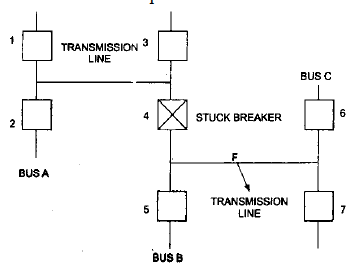Technical Test SSC JE: Electrical Engineering (EE)- 15 - Question 71

In an Anderson bridge, the unknown inductance is measured in term of known

Technical Test SSC JE: Electrical Engineering (EE)- 15 - Question 72

Mho relay is usually employed for the protection of

Technical Test SSC JE: Electrical Engineering (EE)- 15 - Question 73

To measure 5 volts. If one selects a 0 – 100 V range voltmeter which is accurate within + 1%,then the error in this measurement may be up to

Technical Test SSC JE: Electrical Engineering (EE)- 15 - Question 74

The full-load copper loss and iron loss of a transformer are 6,400 W and 5,000 W respectively. The copper loss and iron loss at half load will be, respectively

Technical Test SSC JE: Electrical Engineering (EE)- 15 - Question 75

Curve A and B represent the properties for materials which have

Technical Test SSC JE: Electrical Engineering (EE)- 15 - Question 76

Systematic error are

Technical Test SSC JE: Electrical Engineering (EE)- 15 - Question 77

Vibration galvanometers, tuneable amplifiers and head phones are used in

Technical Test SSC JE: Electrical Engineering (EE)- 15 - Question 78

An impedance relay is a

Technical Test SSC JE: Electrical Engineering (EE)- 15 - Question 79

If the readings of the two wattmeters are equal and positive in two wattmeter method, the load pf in a balanced 3-phase 3-wire circuit will be

Technical Test SSC JE: Electrical Engineering (EE)- 15 - Question 80

The most serious consequence of a major uncleared short-circuit fault could be

Technical Test SSC JE: Electrical Engineering (EE)- 15 - Question 81

A distribution transformer is selected on the basis of

Technical Test SSC JE: Electrical Engineering (EE)- 15 - Question 82

Under the condition of resonance in R-L-C series circuit, the pf of the circuit is

Technical Test SSC JE: Electrical Engineering (EE)- 15 - Question 83

Consider the following statements associated with an energy meter.
1. It is an integrating type instrument.
2. It is an induction type instrument.
3. It uses a permanent magnet for rotation of aluminium disc.
4. it employs a high control torque.
Which of these statements are correct ?

Technical Test SSC JE: Electrical Engineering (EE)- 15 - Question 84

In a.c. circuit, the power is consumed only in

Technical Test SSC JE: Electrical Engineering (EE)- 15 - Question 85

Which portion of the transmission system is more prone to faults ?

Technical Test SSC JE: Electrical Engineering (EE)- 15 - Question 86

An ideal dc generator has a regulation of ____ per cent.

Technical Test SSC JE: Electrical Engineering (EE)- 15 - Question 87

The maximum power that can be distributed in the load in the circuit shown in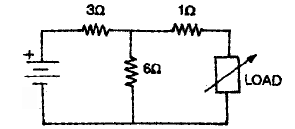Technical Test SSC JE: Electrical Engineering (EE)- 15 - Question 88

Which one of the following is the main cause of creeping in the induction type energy meters ?

Technical Test SSC JE: Electrical Engineering (EE)- 15 - Question 89

The errors introduced by an instrument fall in which category ?

Technical Test SSC JE: Electrical Engineering (EE)- 15 - Question 90

Which portion of the power system is least prone to faults ?

Technical Test SSC JE: Electrical Engineering (EE)- 15 - Question 91

At resonant frequency an R-L-C circuit draws maximum current due to the reason that

Technical Test SSC JE: Electrical Engineering (EE)- 15 - Question 92

Schering bridge can be used to measure which one of the following ?

Technical Test SSC JE: Electrical Engineering (EE)- 15 - Question 93

If an induction type energy meter runs fast, it can be slowed down by

Technical Test SSC JE: Electrical Engineering (EE)- 15 - Question 94

The teeth in the armature of a dc machine are sometimes skewed. This is done to reduce

Technical Test SSC JE: Electrical Engineering (EE)- 15 - Question 95

The maximum short-circuit current occurs in the case of:

Technical Test SSC JE: Electrical Engineering (EE)- 15 - Question 96

The capacitance and loss angle of a given capacitor specimen are best measured by

Technical Test SSC JE: Electrical Engineering (EE)- 15 - Question 97

Impedance relay may be

Technical Test SSC JE: Electrical Engineering (EE)- 15 - Question 98

The most commonly used input signal (s) in control system is/are

Technical Test SSC JE: Electrical Engineering (EE)- 15 - Question 99

Wattless current is possible, only in

Technical Test SSC JE: Electrical Engineering (EE)- 15 - Question 100

For a fault at the terminals of synchronous generator, the fault current is maximum for a

## Mock Test Series for SSC JE Electrical Engineering

2 videos|1 docs|55 tests
 Use Code STAYHOME200 and get INR 200 additional OFF Use Coupon Code
Information about Technical Test SSC JE: Electrical Engineering (EE)- 15 Page
In this test you can find the Exam questions for Technical Test SSC JE: Electrical Engineering (EE)- 15 solved & explained in the simplest way possible. Besides giving Questions and answers for Technical Test SSC JE: Electrical Engineering (EE)- 15, EduRev gives you an ample number of Online tests for practice

## Mock Test Series for SSC JE Electrical Engineering

2 videos|1 docs|55 tests